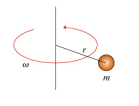# Problem: Consider a particle of mass m = 22.0 kg revolving around an axis with angular speed ω . The perpendicular distance from the particle to the axis is r = 0.750 m.A) Which of the following are units for expressing rotational velocity, commonly denoted by ω? radians per second, degrees per second, revolutions per second

###### FREE Expert Solution

Angular velocity:

$\overline{){\mathbf{\omega }}{\mathbf{=}}\frac{\mathbf{∆}\mathbf{\theta }}{\mathbf{t}}}$

84% (71 ratings)###### Problem Details

Consider a particle of mass m = 22.0 kg revolving around an axis with angular speed ω . The perpendicular distance from the particle to the axis is r = 0.750 m.A) Which of the following are units for expressing rotational velocity, commonly denoted by ω? radians per second, degrees per second, revolutions per second